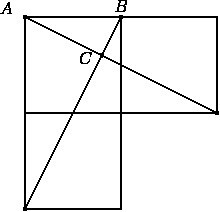# Unit Squares and Triangles

Alignments to Content Standards: G-GPE.B.5 G-GPE.B.4 G-CO.A G-SRT.B.5

Three unit squares and two line segments connecting two pairs of vertices are shown. What is the area of $\triangle ABC$?## IM Commentary

This problem provides an opportunity for a rich application of coordinate geometry. In the first solution, the coordinates of $C$ are calculated explicitly by finding linear equations for the lines containing the sides of the triangles containing $C$. These coordinates then can be used to find the area of $\triangle ABC$ using $\overline{AB}$ as a base. Alternatively $\triangle ABC$ can be shown to be similar to the large right triangles in the picture. Since the large right triangles are each half of a 2 by 1 rectangle their area is readily calculated. If the teacher wishes to emphasize the similarity approach, it may be necessary to provide some guidance. For example, labeling some of the other vertices ($D,E,F$) and having students think about the angles in these triangles: more direct instructions can be provided if needed.

An alternative, in the second solution, for showing that $\overleftrightarrow{AE}$ and $\overleftrightarrow{BF}$ are perpendicular is to use the calculation of slopes in the first solution and the crietrion that two lines (neither of which is horizontal or vertical) are perpendicular when their slopes are inverse reciprocals of one another (which aligns with G-GPE.5).

While the task could serve as a (difficult) assessment task, given the multitude of solution approaches, be wary of using this task to assess any particular standard aligned to this task.

This task was adapted from problem #15 on the 2012 American Mathematics Competition (AMC) 10A Test. For the 2012 AMC 10A, which was taken by 73,703 students, the multiple choice answers for the problem had the following distribution:

 Choice Answer Percentage of Answers (A) ${\frac{1}{6}}$ 6.33 (B)* ${\frac{1}{5}}$ 22.25 (C) ${\frac{2}{9}}$ 8.63 (D) ${\frac{1}{3}}$ 4.29 (E) ${\frac{\sqrt{2}}{4}}$ 10.26 Omit -- 48.22

Of the 73,703 students: 36206, or 49%, were in 10th grade; 25,498 or 35%, were in 9th grade; and the remainder were below than 9th grade.

## Solutions

Solution: 1 Using coordinates to calculate area

We begin by placing the three unit squares on an $x$-$y$ coordinate system so that $A$ is the origin and $B = (1,0)$ as pictured below:Points $D,E,F$ are labelled in the picture. A linear equation whose solutions are the line $\overleftrightarrow{AE}$ is $y=-\frac{1}{2}x$ since $A = (0,0)$ and $E = (2,-1)$ are both solutions to this linear equation. A linear equation whose solutions are the line $\overleftrightarrow{BF}$ is $y=2x-2$ since $B = (1,0)$ and $F = (0,-2)$ are both solutions to this equation. The point $C$ lies on both of these lines and so the coordinates of $C$ simultaneously solve the two equations. If we solve these equations we find $C=\left(\frac{4}{5},-\frac{2}{5}\right)$. Using $\overline{AB}$ as a base, the altitude of the triangle is $\frac{2}{5}$ since $C$ is $\frac{2}{5}$ below the $x$-axis. So the area of $\triangle ABC$ is $$\frac{1}{2} \times 1 \times \frac{2}{5} = \frac{1}{5}.$$

Solution: 2 Rigid motions and similarity

We again begin by placing the three unit squares on an $x$-$y$ coordinate system so that $A$ is the origin and $B = (1,0)$, as pictured below:We know that $\triangle ABF$ is congruent to $\triangle DEA$ by the SAS criterion: $\angle A$ of $\triangle ABF$ and $\angle D$ of $\triangle DEA$ are right angles while $|AF| = |DA| = 2$ and $|AB| = |DE| = 1$. Looking at the two triangles, one way to show this with a rigid motion, would be to rotate $\triangle ADE$ by 90 degrees, counterclockwise, about $A$: a translation by two units downward then completes the congruence. This means that $\overleftrightarrow{BF}$ meets $\overleftrightarrow{AE}$ at a right angle: the rotation of $\overleftrightarrow{AE}$ by 90 degrees meets $\overleftrightarrow{AE}$ in a right angle at $A$. The translation two units down moves the point of intersection to $C$, but does not affect the angle of intersection. Alternatively, $\angle ABC$ is the same as angle of $ABF$ which is congruent to angle $DEA$. We know $m(\angle DEA) + m(\angle EAD) = 90$ and so $m(\angle ABC) + m(\angle CAB) = 90$ and $\angle BCA$ must be a right angle.

Next we claim that $\triangle ACB$ is similar to $\triangle FAB$. To see why, notice that $\angle A$ is congruent to $\angle F$ because $\triangle DEA$ is congruent ot $\triangle ABF$. We also know that $\angle ACB$ and $\angle FAB$ are right angles (the latter because it is made by coordinate grid lines). By the AA criterion for similarity. $\triangle ACB$ is similar to $\triangle FAB$. We have $|AB|=1$ and $$|BF|=\sqrt{|AB|^2 + |AF|^2} = \sqrt{5}.$$ So the scale factor used to get from $\triangle ACB$ to $\triangle FAB$ is $\sqrt{5}$. The area of $\triangle FAB$ is $1$ since it is half of a two by one rectangle. Since area grows/shrinks by the square of the scale factor, we know that that $\triangle FAB$ has 5 times the area of $\triangle ACB$. We conclude that the ratio of the area of $\triangle ACB$ to that the area of $\triangle ACB$ is $\frac{1}{5}$.